# NEET Physics Magnetism and Matter Questions Solved

A bar magnet of length l and magnetic dipole moment M is bent to form an arc which subtends an angle of $120°$ at centre. The new magnetic dipole moment will be

1.  $\frac{3\mathrm{M}}{2\mathrm{\pi }}$

2.  $\frac{3\sqrt{3}\mathrm{M}}{2\mathrm{\pi }}$

3.  $\frac{3\mathrm{M}}{\mathrm{\pi }}$

4.  $\frac{2\mathrm{M}}{\mathrm{\pi }}$

Concept Videos :-

#3 | Magnetic Dipole Moment & Field due to Bar Magnet
#4 | Superposition of Magnetic Field due to Bar Magnet
#5 | Field due to Short Bar Magnet
#6 | Torque Acting on Bar Magnet in Uniform Field
#7 | Work Done in Rotating Dipole in Field & PE
#8 | Oscillations of Bar Magnet in Uniform Field
#9 | Solenoid as Equivalent Bar Magnet

Concept Questions :-

Bar magnet
Complete Question Bank + Test Series
Complete Question Bank

Difficulty Level:

Concept Videos :-

#10 | Earth's Magnetism
#11 | Apparent & True Dip
#12 | Neutral points
#13 | Comparison of Magnetic Field of Earth at Two Points
#14 | Variation of Earth's Magnetic Field

Concept Questions :-

Earth's magnetism
Complete Question Bank + Test Series
Complete Question Bank

Difficulty Level: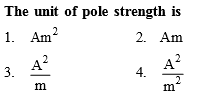Concept Videos :-

#3 | Magnetic Dipole Moment & Field due to Bar Magnet
#4 | Superposition of Magnetic Field due to Bar Magnet
#5 | Field due to Short Bar Magnet
#6 | Torque Acting on Bar Magnet in Uniform Field
#7 | Work Done in Rotating Dipole in Field & PE
#8 | Oscillations of Bar Magnet in Uniform Field
#9 | Solenoid as Equivalent Bar Magnet

Concept Questions :-

Bar magnet
Complete Question Bank + Test Series
Complete Question Bank

Difficulty Level: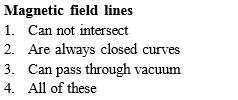Concept Videos :-

#2 | Magnetic Field & Magnetic Field Lines

Concept Questions :-

Magnetic field and field lines
Complete Question Bank + Test Series
Complete Question Bank

Difficulty Level: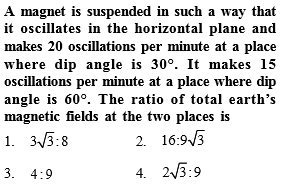Concept Videos :-

#10 | Earth's Magnetism
#11 | Apparent & True Dip
#12 | Neutral points
#13 | Comparison of Magnetic Field of Earth at Two Points
#14 | Variation of Earth's Magnetic Field

Concept Questions :-

Earth's magnetism
Complete Question Bank + Test Series
Complete Question Bank

Difficulty Level:

1.

2.

3.

4.  ${\mathrm{tan}}^{-1}\left(\frac{2}{\sqrt{3}}\right)$

Concept Videos :-

#10 | Earth's Magnetism
#11 | Apparent & True Dip
#12 | Neutral points
#13 | Comparison of Magnetic Field of Earth at Two Points
#14 | Variation of Earth's Magnetic Field

Concept Questions :-

Earth's magnetism
Complete Question Bank + Test Series
Complete Question Bank

Difficulty Level: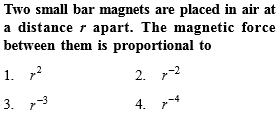Concept Videos :-

#1 | Analogy b/w Electrostatics & Magnetostatics

Concept Questions :-

Analogy between electrostatics and magnetostatics
Complete Question Bank + Test Series
Complete Question Bank

Difficulty Level: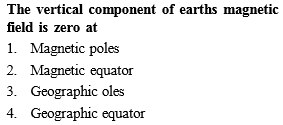Concept Videos :-

#10 | Earth's Magnetism
#11 | Apparent & True Dip
#12 | Neutral points
#13 | Comparison of Magnetic Field of Earth at Two Points
#14 | Variation of Earth's Magnetic Field

Concept Questions :-

Earth's magnetism
Complete Question Bank + Test Series
Complete Question Bank

Difficulty Level: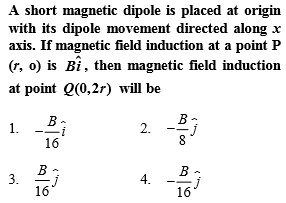Concept Videos :-

#1 | Analogy b/w Electrostatics & Magnetostatics

Concept Questions :-

Analogy between electrostatics and magnetostatics
Complete Question Bank + Test Series
Complete Question Bank

Difficulty Level:

The magnetic field at a point x on the axis of a small bar magnet is equal to the field at a point y on the equator of the same magnet. The ratio of the distances of x and y from the centre of the magnet is
(a) ${2}^{-3}$

(b) ${2}^{-1}{3}}$

(c) ${2}^{3}$

(d) ${2}^{1}{3}}$

Concept Videos :-

#3 | Magnetic Dipole Moment & Field due to Bar Magnet
#4 | Superposition of Magnetic Field due to Bar Magnet
#5 | Field due to Short Bar Magnet
#6 | Torque Acting on Bar Magnet in Uniform Field
#7 | Work Done in Rotating Dipole in Field & PE
#8 | Oscillations of Bar Magnet in Uniform Field
#9 | Solenoid as Equivalent Bar Magnet

Concept Questions :-

Bar magnet